# Present Value of an Ordinary Annuity (PVOA)

Ordinary annuities are also known as annuities in arrears. These annuities are characterized by recurring, identical, cash payment amounts (payments, receipts, rents) at the end of each equal period. The following timeline illustrates a 5-year ordinary annuity with payments of \$100 occurring at the end of each year: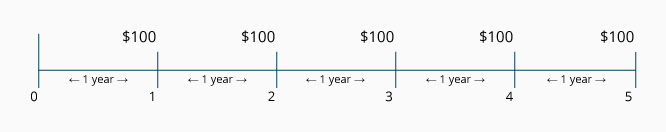Examples of ordinary annuities include:

• An automobile loan taken out on May 25, 2023 requires a monthly payment of \$300 for 48 months beginning on June 25, 2023.

• A mortgage loan dated June 1, 2023 requires a payment of \$943 on the first day of each month for 180 months beginning on July 1.

• The interest payments on a bond payable issued on May 1, 2023 requires \$10,000 of interest be paid every November 1 and May 1 for the life of the bond.

• A commitment made on January 1, 2023 requires \$1,000 be paid each December 31 for five years.

### Visualizing the Present Value of an Ordinary Annuity (PVOA)

In order to calculate the present value of an ordinary annuity (PVOA), you need to know the other four components mentioned above:

• Amount of each identical cash payment
• Time between the identical cash payments
• Number of periods that the payments will occur
• Interest rate used for discounting the series of payments

Let's assume that on May 1, 2023, you are asked to determine the present value of a series of \$1,000 receipts. The receipts will be received each year on May 1 beginning in 2024 and ending in 2028. The appropriate rate for discounting the \$1,000 amounts is 12% per year. If we insert the known and unknown amounts onto a timeline, we minimize the potential for making an error: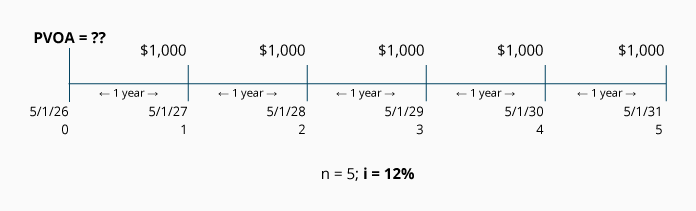### Visualizing the Recurring Identical Payments (PMT)

Often we know the present value, the number of payments, and the interest rate, but we do not know the amount of the recurring payments. For example, we may want to calculate the amount of a monthly loan payment if we borrowed \$25,000 for five years; or a recent retiree may want to know how much could be withdrawn every six months for twenty years from a fixed account earning 5% interest.

Let's look at an example. Assume that on June 1, 2023, your savings account has a present value of \$8,530 and it earns interest at the semiannual rate of 3% (approximately 6% per year). Beginning on December 1, 2023, you want to withdraw an equal amount of cash each December 1 and June 1 so that at the end of 5 years the account balance is zero. What will be the amount of your semiannual withdrawals?

Let's construct a timeline to help determine the amount of each semiannual withdrawal or payment (PMT):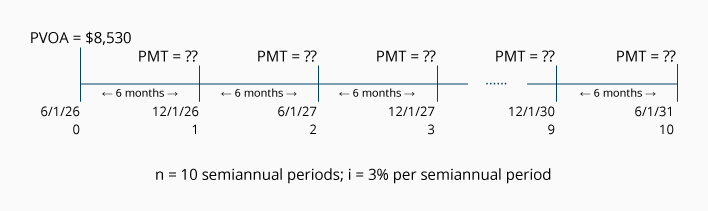Using an alternative scenario, this timeline could be used to describe a present debt amount of \$8,530 that needs to be paid off with 10 semiannual loan payments and a loan interest rate of 6% per year (3% semiannually).

### Visualizing the Number of Periods (n)

In some instances, you may need to determine the number of payments in an ordinary annuity. For example, for how many months could you withdraw \$100 from an investment account with a present value of \$2,581, if the fund earns 12% per year compounded monthly. The fund begins on June 1, 2023 and the first monthly withdrawal takes place on July 1, 2023.

The following timeline summarizes the information: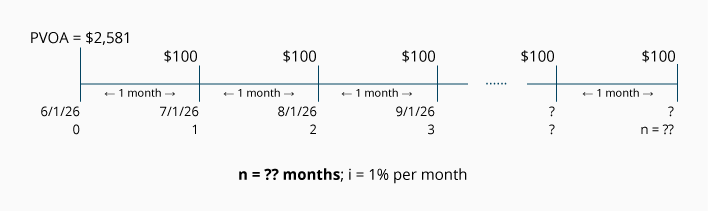Using an alternative scenario, this timeline could depict the number of periods it would take to pay off a present loan balance of \$2,581 with an interest rate of 1% per month and monthly payments of \$100.

### Visualizing the Interest Rate (i)

The interest rate (i) could be the interest rate in a loan, but it could also be a company's cost of capital, a targeted rate the company must earn on its investment, or it could be the actual rate of return being earned.

In some situations, you know the present value of an ordinary annuity, the recurring identical payment amounts, the time interval between the payments, and the length of the annuity. You are asked to determine the interest rate (i) or the rate of return in the annuity.

To illustrate, let's assume that an annuity consists of four payments of \$100 each and they will occur on July 1 of each year beginning in 2024. The present value on July 1, 2023 is \$317. You are asked to determine the interest rate (or rate of return) involved in this arrangement. To aid in visualizing this annuity, you prepare the following timeline: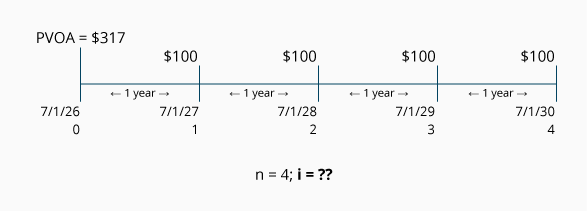Using an alternative scenario, this timeline could be used to depict a situation in which your company has the choice of purchasing an item today for \$317, or paying \$100 in four annual installments (with the first payment due in one year). You are asked to determine the interest rate that your company would be paying under the four-payment option.